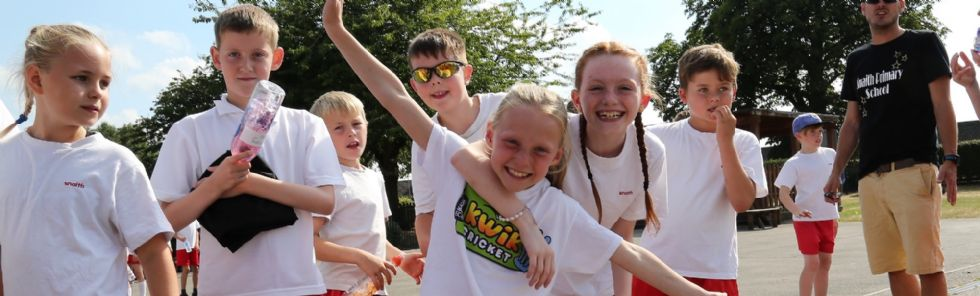;••# Big Maths

We use Big Maths in our class to help us understand the steps of our learning, track our progress and develop as mathematicians.

As part of our Big Maths Scheme children are given number sentences to learn off by heart each week. The children are required to learn the facts and their corresponding switchers and fact families. Each week your child is given a Big Maths Beat That Test, where they are given a set time to answer as many facts as possible.

#### Step 2

##### Step 4
1+1=2 3+3=6 2+3=5 1+9=10
2+2=4 4+4=8 2+1=3 2+8=10
5+5=10   3+7=10

4+6=10

##### Step 7
4+2=6 6+6=12 8+3=11 4x10=40
5+2=7 7+7=14 9+3=12 5x10=50
6+2=8 8+8=16 7+4=11 6x10=60
7+2=9 9+9=18 8+4=12 7x10=70
9+2=11   9+4= 13 8x10=80
4+3=7   1x10=10 9x10=90
5+3=8   2x10=20 10x10=100
6+3=9   3x10=30

##### Step 9

5+4= 9 4x5=20 5+7 = 12 3x2=6
5+6=11 5x5=25 5+8= 13 4x2=8
6+7=13 6x5=30 5+9=14 5x2=10
8+7=15 7x5=35 6+8= 14 6x2=12
8+9=17 8x5=40 6+9= 15 7x2=14
1x5=5 9x5=45 7+9 = 16 8x2=16
2x5=10 10x5=50 1x2=2 9x2=18
3x5=15   2x2=4 10x2=20

##### Step 11

1x3=3 6x3=18 1x4=4 6x4=24
2x3=6 7x3=21 2x4=8 7x4=28
3x3=9 8x3=24 3x4=12 8x4=32
4x3=12 9x3=27 4x4=16 9x4=36
5x3=15 10x3=30 5x4=20 10x4=40